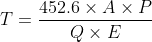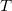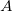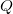# 1" Application Time

Use this form to calculate the irrigation time to apply one inch of water with a center pivot. Find the irrigated area underneath the center pivot by using the pivot acreage calculator. Learn more about the units used on this page.

Flow Rate:
Percent of Full Circle (for wipers):
%
Irrigation Application Efficiency:
%
Time:

## The Equation

This calculator uses this formula to determine the 1" application timeWhere:= Time it takes to complete a cycle while applying 1" (hrs)= Irrigated Area (acres)= Flow rate at the pivot (gpm)= Percent of full circle being irrigated (as a decimal)= Irrigation efficiency (as a decimal)

WSU Prosser - IAREC, 24106 N Bunn Rd, Prosser WA 99350-8694, 509-786-2226. Contact Us